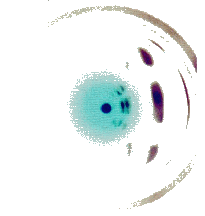Chris Thornton

# Bayes rule again

Given evidence E and some conclusion C, it's always the case that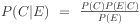We can plug any values we like into this formula to infer the probability of the conclusion given the evidence.

# Lecture attendance example

So, from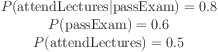we can use Bayes rule to calculate probability of passing the exam: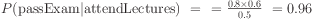The probability of passing the exam given you attend lectures is 0.96.

# Complex Bayesian reasoning

Bayes' rule provides a single step of probabilistic backwards reasoning.

This works for simple scenarios, e.g., where we have a lot probabilities relating diseases to symptoms, and want a rule that produces a diagnosis from the symptoms shown.

But in more complex cases, we may have a networks or chain of probabilistic relationships to deal with.

For example,

```  cheapMoney => consumerBorrowing => highDemand => inflation
```
How do we represent and perform inference with complex chains of this sort?

# Probabilistic representation

Let's say we want to work out if it's going to be humid tomorrow.

We have a tangle of relationships to take into account. Sunshine increases the humidity but so does rain and temperature, both of which are themselves affected by sunshine.

• Represent each element of the domain as a variable which takes certain values (e.g., sun=yes, sun=no).

• Represent the relationships between variables in terms of conditional probabilities, e.g., probabilities like P(temp=high|sun=yes) = 0.8, P(temp=high|sun=no) = 0.2, P(rain=yes|temp=low) = 0.6 etc.

# Apply the laws of probability

To find out if it's going to be humid, start with some variables that we know the value of and work forwards, establishing the probability distribution on one variable by taking into account all its conditional probabilities and all the (distributions on) all the variables which those probabilities depend on.

This is also known as forwards propagation.

# Bayesian network

Draw out the network of conditional relationships and annotate nodes with the CPTs (conditional probability tables).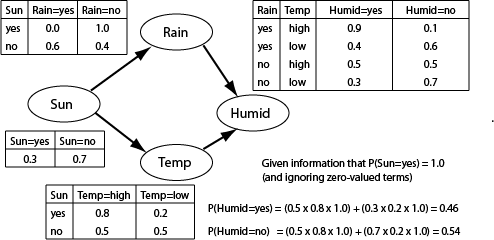# Reasoning as propagation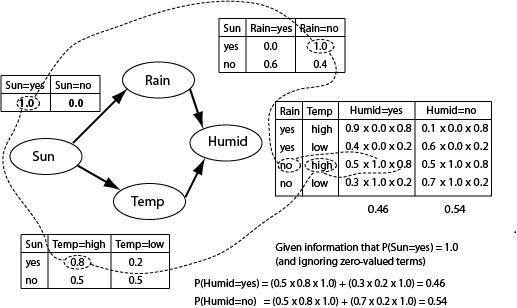# Terminology and assumptions

In Bayesian networks, any variable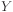which has a direct influence on variableis said to be's parent.

An arrow points from parenttoVariableis then said to be's child, whileand all's children are's descendants.

When reasoning is done using probability propagation, the assumption is made that two variables are conditionally independent of all non-descendants given their parents.

This is another way of saying that variables are only influenced by their parents.

# Top-down/forwards propagation

To find the probability of the ith value of variable, use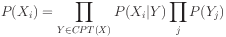This defines the distribution onrecursively. Each value is obtained by iterating over the combinations of parental values taking the product of the combination's probability and the probability of the value which is conditional on the combination.

# Termination

Termination is achieved by providing a non-conditional distribution for some root variable.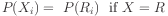Note that distributions must sum to 1 (so normalization may be required).

# How well does it work?

Reasoning using Bayesian nets works perfectly in the sense that probabililies are consistently propagated.

But depending on how variables are related, we can easily end up with very uncertain conclusions.

The key factor which affects performance is the level of uncertainty we have about conditioned variables.

This is the termed equivocation.

# Equivocation formula

To calculate the equivocation of a conditioned variable relative to a conditioning variable, derive the weighted average of the uncertainties (entropies) of conditional distributions.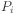is the conditioned probability of the ith value of the conditioned variable, and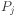is the probability of the conditioning value.

# Equivocation as a weighted uncertainty sum

Equivocation is really just a weighted sum of the uncertainties of the conditional distributions.

Other things being equal, higher equivocation will mean less successful Bayesian reasoning, i.e., less certain conclusions.

.

# Summary

• Bayes rule again

• Probabilistic representation

• Use of Bayesian networks

• Reasoning as propagation

• Top-down propagation

• Equivocation

# Questions

• Let's say the university communicates your degree result to you using either a tick or a cross. What is the level of equivocation?

# Exercises

• Use Bayes' rule to work out P(east|sun) given that P(sun)= 0.3, P(east)=0.4 and P(sun|east)=0.6.

• Use the frequency interpretation of probability to explain why Bayes rule works.